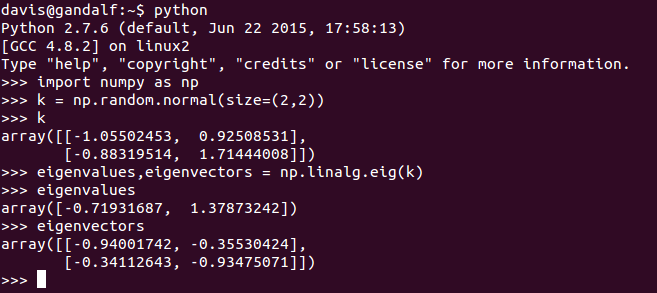# Linear Algebra

## my old friend

Posted by on January 31, 2016 · 1 min read

As part of explorations into machine learning, I’ve been brushing up on computer science basics starting with linear algebra. Nice to see good old eigenvectors again after so many years.

An eigenvector of a square matrix $A$ is a non-zero vector $v$ such that multiplication by $A$ alters only the scale of $v$:

$\mathrm{Av}=\mathrm{\lambda v}$

The scalar $\lambda$ is known as the eigenvalue corresponding to this eigenvector.

Excerpted from Chapter 2 of Deep Learning by Ian Goodfellow, Yoshua Bengio and Aaron Courville.

## Practical Use?

So, why are eigenvectors important? Well, they are used in singular value decomposition, which can be applied in principal component analysis as mentioned in this podcast on machine learning fundamentals.

## Example Code

Here’s a quick way of calculating eigenvalues and eigenvectors in Python using the numpy library:

import numpy as np
k = np.random.normal(size=(2,2))
eigenvalues,eigenvectors = np.linalg.eig(k)


with output shown in this interactive session: